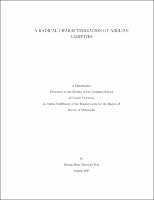## A Radical Characterization of Abelian Varieties

 dc.contributor.author Hui, Heung Shan Theodore dc.contributor.chair Zywina, David J. dc.contributor.chair Ramakrishna, Ravi Kumar dc.contributor.committeeMember Speh, Birgit E M dc.date.accessioned 2018-04-26T14:18:17Z dc.date.available 2018-04-26T14:18:17Z dc.date.issued 2017-08-30 dc.description.abstract Let $A$ be a square-free abelian variety defined over a number field $K$. Let $S$ be a density one set of prime ideals $\p$ of $\mathcal{O}_K$. A famous theorem of Faltings says that the Frobenius polynomials $P_{A,\p}(x)$ for $\p\in S$ determine $A$ up to isogeny. We show that the prime factors of $|A(\FF_\p)|=P_{A,\p}(1)$ for $\p\in S$ also determine $A$ up to isogeny over an explicit finite extension of $K$. The proof relies on understanding the $\ell$-adic monodromy groups which come from the $\ell$-adic Galois representations of $A$, and the absolute Weyl group action on their weights. We also show that there exists an explicit integer $e\geq 1$ such that after replacing $K$ by a suitable finite extension, the Frobenius polynomials of $A$ at $\p$ must equal to the $e$-th power of a separable polynomial for a density one set of prime ideals $\p\subseteq\mathcal{O}_K$. dc.identifier.doi https://doi.org/10.7298/X41V5C30 dc.identifier.other Hui_cornellgrad_0058F_10398 dc.identifier.other http://dissertations.umi.com/cornellgrad:10398 dc.identifier.other bibid: 10361681 dc.identifier.uri https://hdl.handle.net/1813/57004 dc.language.iso en_US dc.subject radical dc.subject Galois representations dc.subject Mathematics dc.subject abelian varieties dc.subject monodromy groups dc.title A Radical Characterization of Abelian Varieties dc.type dissertation or thesis dcterms.license https://hdl.handle.net/1813/59810 thesis.degree.discipline Mathematics thesis.degree.grantor Cornell University thesis.degree.level Doctor of Philosophy thesis.degree.name Ph. D., Mathematics
##### Original bundle
Now showing 1 - 1 of 1